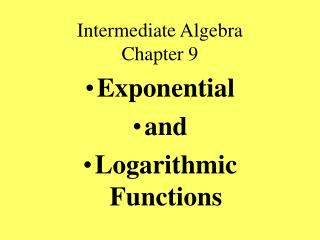DownloadDownload PresentationIntermediate Algebra Chapter 9

# Intermediate Algebra Chapter 9

Download Presentation## Intermediate Algebra Chapter 9

- - - - - - - - - - - - - - - - - - - - - - - - - - - E N D - - - - - - - - - - - - - - - - - - - - - - - - - - -
##### Presentation Transcript

1. Intermediate AlgebraChapter 9 • Exponential • and • Logarithmic Functions

2. Intermediate Algebra 9.1-9.2 • Review of Functions

3. Def: Relation • A relation is a set of ordered pairs. • Designated by: • Listing • Graphs • Tables • Algebraic equation • Picture • Sentence

4. Def: Function • A function is a set of ordered pairs in which no two different ordered pairs have the same first component. • Vertical line test – used to determine whether a graph represents a function.

5. Defs: domain and range • Domain: The set of first components of a relation. • Range: The set of second components of a relation

6. Examples of Relations:

7. Objectives • Determine the domain, range of relations. • Determine if relation is a function.

8. Intermediate Algebra 9.2 • Inverse Functions

9. Inverse of a function • The inverse of a function is determined by interchanging the domain and the range of the original function. • The inverse of a function is not necessarily a function. • Designated by • and read f inverse

10. One-to-One function • Def: A function is a one-to-one function if no two different ordered pairs have the same second coordinate.

11. Horizontal Line Test • A function is a one-to-one function if and only if no horizontal line intersects the graph of the function at more than one point.

12. Inverse of a function

13. Inverse of function

14. Objectives: • Determine the inverse of a function whose ordered pairs are listed. • Determine if a function is one to one.

15. Intermediate Algebra 9.3 • Exponential Functions

16. Michael Crichton – The Andromeda Strain (1971) • The mathematics of uncontrolled growth are frightening. A single cell of the bacterium E. coli would, under ideal circumstances, divide every twenty minutes. It this way it can be shown that in a single day, one cell of E. coli could produce a super-colony equal in size and weight to the entire planet Earth.”

17. Definition of Exponential Function • If b>0 and b not equal to 1 and x is any real number, an exponential function is written as

18. Graphs-Determine domain, range, function, 1-1, x intercepts, y intercepts, asymptotes

19. Graphs-Determine domain, range, function, 1-1, x intercepts, y intercepts, asymptotes

20. Growth and Decay • Growth: if b > 1 • Decay: if 0 < b < 1

21. Properties of graphs of exponential functions • Function and 1 to 1 • y intercept is (0,1) and no x intercept(s) • Domain is all real numbers • Range is {y|y>0} • Graph approaches but does not touch x axis – x axis is asymptote • Growth or decay determined by base

22. Natural Base e

23. Calculator Keys • Second function of divide • Second function of LN (left side)

24. Property of equivalent exponents • For b>0 and b not equal to 1

25. Compound Interest • A= amount P = Principal t = time • r = rate per year • n = number of times compounded

26. Compound interest problem • Find the accumulated amount in an account if \$5,000 is deposited at 6% compounded quarterly for 10 years.

27. Objectives: • Determine and graph exponential functions. • Use the natural base e • Use the compound interest formula.

28. Dwight Eisenhower – American President • “Pessimism never won any battle.”

29. Intermediate Algebra 9.4,9.5,9.6 • Logarithmic Functions

30. Definition: Logarithmic Function • For x > 0, b > 0 and b not equal to 1 toe logarithm of x with base b is defined by the following:

31. Properties of Logarithmic Function • Domain:{x|x>0} • Range: all real numbers • x intercept: (1,0) • No y intercept • Approaches y axis as vertical asymptote • Base determines shape.

32. Shape of logarithmic graphs • For b > 1, the graph rises from left to right. • For 0 < b < 1, the graphs falls from left to right.

33. Calculator Keys • [LOG] • [LN]

34. Objective: • Determine the common log or natural log of any number in the domain of the logarithmic function.

35. Change of Base Formula • For x > 0 for any positive bases a and b

36. Problem: change of base

37. Objective • Use the change of base formula to determine an approximation to the logarithm of a number when the base is not 10 or e.

38. Intermediate Algebra 10.5 • Properties • of • Logarithms

39. Basic Properties of logarithms

40. For x>0, y>0, b>0 and b not 1Product rule of Logarithms

41. For x>0, y>0, b>0 and b not 1Quotient rule for Logarithms

42. For x>0, y>0, b>0 and b not 1Power rule for Logarithms

43. Objectives: • Apply the product, quotient, and power properties of logarithms. • Combine and Expand logarithmic expressions

44. Theorems summary Logarithms:

45. Norman Vincent Peale • “Believe it is possible to solve your problem. Tremendous things happen to the believer. So believe the answer will come. It will.”

46. Intermediate Algebra 9.7 • Exponential • and • Logarithmic • Equations

47. Objective: • Solve equations that have variables as exponents.

48. Exponential equation